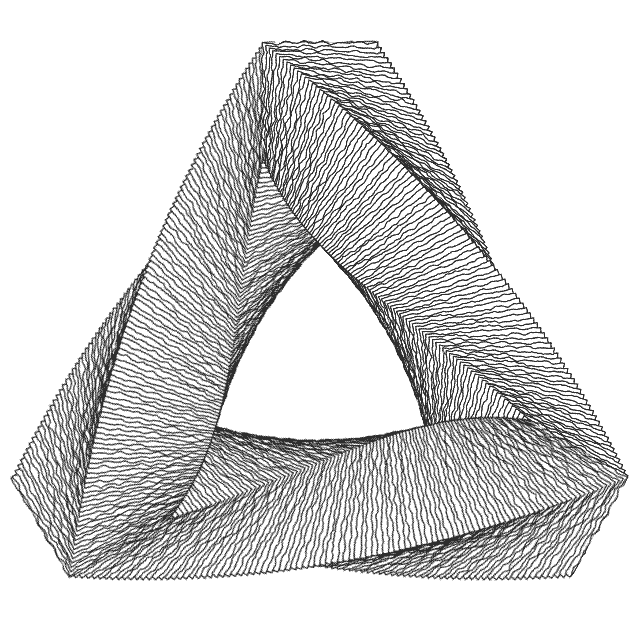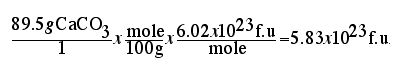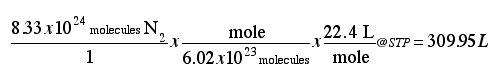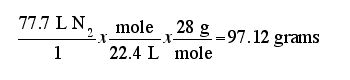#Ralph & Bev ShockThe Mole Concept:

The mole is the SI unit for an amount of substance, just like dozen means 12 things or a gross means 144 things, the mole is 6.02x1023 things. This very large number is necessary to handle convenient amounts of matter in the range of atoms and molecules. This number is called Avogadro's number in honor Amedeo Avogadro di Quarenga an Italian chemist (1776-1856).

It is sometimes more helpful to see the usefulness of the mole as a practical way of measuring out chemical substances than just a very large number of things.

Since atomic masses are relative quantities, the atomic mass in grams of one element contains the same number of atoms in the atomic mass of a different element. This number of atoms is 6.02x1023 atoms. This means that a gram equivalent mass of carbon 12.01 grams contains the same number of atoms as 16.00 grams of oxygen. Note that these numbers represent their atomic masses represented in grams. Therefore, 12.01 grams of carbon equals 1mole of particles (atoms) and 16.00 grams of oxygen equals 1 mole of particles(atoms). Since most of the problems we solve are done as factor-label problems it is most useful to represent these relationships as ratios.

This gives us a way to measure out grams of chemical substances that relate to the ratio of atoms in chemical formulas and chemical equations.

Example: If we measure out 12.0 grams of carbon and 16.00 grams if oxygen the relationship we get is:

12.01g/mole for carbon and 16.00 g/mole for oxygen . These ratios are referred to as their gram atomic masses. Note the relationship of Adogadro's number to the mole:

6.02x1023 particles/mole.

Using factor-label we can prove there are 6.02x1023 particles in a mole of oxygen.

(16.00g/mole)x (mole/6.02x1023 atoms)= 16.00g/6.02x1023 atoms. Therefore 16.00 grams of atomic oxygen=6.02x1023 atoms of oxygen.

So we can see how useful the mole is for measuring out convenient amounts of substance.

This means that a gram equivalent mass of any element is equal to a mole of that element.

Since elements always combine in simple whole number ratios to form compounds, the mole concept can be applied to compounds just by determining the gram formula mass of a substance. Since water consists of 2 hydrogen atoms bonded to 1 oxygen atom (H2O) and the mass of hydrogen is 1.01 and the mass of oxygen is 16.00 the formula mass is 18.02 (2-Hx1.01)+(1-O x16.00)=18.02. This means that 18.02 grams of water is equal to one mole of water molecules and that 18.02 grams of water contains 6.02x1023 molecules of water.

This brings us to the most important concept of the mole. A chemical formula may be interpreted in two ways. Example: The formula for water can be interpreted as 2 hydrogen atoms bonded to one oxygen atom or it can be interpreted as 2 moles of hydrogen atoms bonded to one mole of oxygen atoms. We will see later the ramifications of this for determining the amount of reactants needed to produce a desired amount of product in chemical reactions.

The preceding discussion of the mole has already shown us two useful relationships for solving mole problems:

g/mole where the grams is determined from the atomic mass of an element or the formula mass of a command.

6.02x1023 particles/mole where the particle is an atom for elements, molecules for molecular compound and formula units (f.u.) for ionic compounds.

A third relationship is applied to gaseous substances at 0 ºC and 1 atmosphere of pressure. These conditions are called Standard Temperature and Pressure or STP for short. This means one mole of any gas (no matter its formula) will occupy 22.4 liters of volume at these conditions. Therefore:

22.4 L of gas @STP = 1mole

i.e. 22.4Lgas/mole @STP

We now have all the relationships (ratios) necessary to solve mole problems using the factor label method. Below is a stepwise procedure for solving mole problems.

Follow these directions explicitly to solve the following problems.
1. Record what you are to find: (Give the name of the quantity, the symbol for the name, and the probable units.)

2. Record each value given: (Give the name of the quantity, the symbol for the name, and the value with units.)

3. Record other values: which may be needed to solve the problem. (Give the name of the quantity, the symbol for the name, and the value with units.)

4. If you will use a formula to solve the problem

(a) Write down the basic formula,

(b) Solve the formula algebraically for the unknown,

(c) Substitute the values into the solved formula,

(d) Solve for the numerical answer.

5. Show all work for every step of the calculations. (Ex. H2O = (2 X1) + (1 X 16) = 18.

6. Check your work to see that the answer is logical and that the units work algebraically to give the final desired units.

EXAMPLE: What volume will be occupied by 4.00 g of oxygen gas at STP?
( or Conversion factors)
4.00 g x 1 mol/32.0g x 22.4 L/mole = 2.80 L

Remember there are three conversion factors used to solve mole problems:

grams/mole where the grams is calculated from the formula mass or atomic mass

22.4 L/mole at STP (standard temperature and pressure 0.0 ºC and 1 atmosphere pressure)

6.022 x 1023 particles/mole Where the particles are atoms for elements, molecules for molecular compound, formula units for ionic compound.

One or more conversion factor can be used in a problem: In this problem we are determining the number of formula units in 89.5 g of CaCO3. Therefore both the factor for g/mole and 6.02x1023 particles/mole is used.How many liter would 8.33x1024 molecules of nitrogen gas occupy at STP?How many grams are 77.7 liters of dinitrogen monoxide at STP?Percent Composition Problems:

Percent composition is the percent contribution by mass of each element in a compound. This mean that you must determine the total formula mass and then divide the mass contribution of each element in the formula by the total mass times 100 percent.

Example: the percent composition for Calcium phosphate is:
1. determine the correct formula: Ca3(PO4)2 therefore it has:

3 calcium atoms at 40 amus each = 120 amus contributed by calcium
2 phosphorus atoms at 31 amus each= 61 amus contributed by phosphorus
8 oxygen atoms at 16 amus each = 128 amus contributed by oxygen
The total formula mass is 120+61+128 = 309

percent composition is (120/309)*100%= 38.8% calcium
(61/309)*100%= 19.7 % phosphorus
(128/309)*100%= 41.5 % oxygen

This information can be useful for solving other problems.

Example:

Suppose you have a 155.5 g sample of Limestone that is 85.0% Calcium Carbonate by mass and you need to know how many grams are just calcium.

1st. Determine the grams of pure Calcium Carbonate you have by multiplying the mass of the sample times the fraction that is pure Calcium Carbonate:

155.5g x 0.850 = 132.175 g of pure Calcium Carbonate.

2nd. Determine the grams that are just Calcium by multiplying the mass of the pure Calcium Carbonate by the fraction that is just Calcium

132.175 g CaCO3 x 0.388 = 51.3 grams Ca.

Empirical Formula Problems:

An empirical Formula is the lowest whole number ratio of atoms in a chemical formula. For ionic compounds the chemical formula is always the empirical formula.

Example: For Cesium Phosphate:Cs3PO4 the ratio of atoms in this formula is the lowest whole number ratio; 3 cesium atoms to 1 phosphorous atom to 4 oxygen atoms.

For molecular compound the chemical formula may or may not be an empirical formula.

Example: For water H2O this is the ratio of atoms that represent a molecule of water and it is the lowest whole number ratio. On the other hand C6H12O6 is the molecular formula for a sugar. This formula represents the ratio of atoms for one molecule of sugar. However, this is not the lowest whole number ratio because it can be divided by 6 to give us CH2O. This is the empirical formula.

Empirical formulas can be determined from percent composition data because if we assume we have a 100 grams sample of a substance then the grams can be converted into moles and as stated earlier that a chemical formula can be interpreted as a ratio of atoms or a ratio of moles. The mole-mole ratios of elements in a compound represent the ratio of atoms in an empirical formula.

Steps for solving empirical formula problems:

1. If the elements that make up the compound are given in percents.
Assume that you have a 100 grams sample. Then the percents are equivalent to grams.

2. Take the grams of each element and divide by the gram atomic mass to get the moles of each element.

3. Divide all other elements by the element with the least number of moles to get the mole-mole ratios.
The mole mole ratio represents the ration of atoms to each other in the formula.
If the mole -mole ratios have fractional ratios that can't be rounded to a whole number because the first decimal place is greater that one, you must multiply the ratios by some multiple to get a whole number ratio.

4. If the problem is a molecular formula problem then additional information must be contained in the problem statement for the gram molecular mass.
The empirical mass is calculated from the empirical formula as determined from steps 1-3. then the empirical mass is divided into the molecular mass to get the ratio between the empirical formula and molecular formula.

Example: If the percent composition of a compound is:

percent composition is:

38.8% calcium =38.8 gram for a 100.0 gram sample

19.7 % phosphorus = 19.7 grams for a 100.0 gram sample

41.5 % oxygen = 41.5 grams for a 100.0 gram sample

What is the empirical formula?

38.8 g / 40.01g/mole= 0.968 moles Ca
19.7 g/30.97g/mole= 0.636 moles P
41.5 g/16.00g/mole= 2.594 moles O
find the smallest number of moles: Which is phosphorus : 0.636

Of course 0.636moles P/0.636 moles P = 1mole P to 1mole P

Divide this into the other moles to get mole-mole ratios

0.969 moles Ca/ 0.635 moles P = 1.5

2.594 moles O / 0.636 moles P = 4

therefore the ratio is 1.5 moles Ca : 4 moles O

Since Ca is not a whole number each ratio must be multiplied by whole number to give us whole number ratios. In this case : 2. Therefore the ratios are 3 Ca to 2 P to 8 oxygen.

This would lead us to an initial empirical formula of: Ca3P2O8 but this does not look like a familiar compound. Since this compound contains more that 3 elements and a metal it has to have a polyatomic ion. therefore we look for a polyatomic ion containing phosphorous and oxygen. The choices are the phosphate ion (PO4-3) and the phosphite ion (PO3-3), because the ratio of phosphorous to oxygen in the initial formula is 1:4 then the only possible formula is:

Ca3(PO4)2

Empirical Formula Problems With Hydrated Crystals:

Ionic compound dissociate in water into their respective ions. This means when an ionic compound such as copper (II) sulfate is dissolved in water the polarity of the water molecules causes the ions to separate. This separation causes the ions to be surround by a sphere of water molecules that are attracted to the charges on the ions. This sphere is called a solvation sphere or sphere of hydration. When the bulk of the water is slowly evaporated away the water molecules in the sphere of hydration become more and more strongly attracted to the ions. When the solution is saturated crystals begin to form. The water molecules become trapped in the crystalline structure in very specific ratios. For Copper (II) Sulfate , 5 water molecules become trapped for every formula unit. The Copper (II) Sulfate is said to be hydrated and its formula is represented as: CuSO4 * 5H2O and the name is :Copper(II)Sulfate * Pentahydrate.

When a percent composition is given for a hydrated crystal it includes the percents for the hydrogen and oxygen from the water.

In order to solve an empirical formula problem for a hydrated crystal the overall percent composition must be given and the percent of water in the analysis.

Lets assume we don't already know the formula for hydrated Copper(II)Sulfate and were given the following empirical formula problem.

Analysis of a compound gives us the following percentages: 25.97% Cu, 13.10% S, 58.87% O and 4.12% H. What is the empirical formula if the compound contained 36.84 % water of hydration.

Step 1: Assume a 100g sample therefore the percentages are equivalent to grams.

Step 2: Convert Grams to moles using the gram atomic masses for the elements and the formula mass of water.

25.97g Cu x mole/63.55 g = 0.409 moles of Cu

13.10 g S x mole/32.06 g = 0.409 moles of S

58.87 g O x mole/16.00 g =3.68 moles 0 ( includes O from water)

4.12 g H x mole/1.01 g = 4.08 moles H ( includes H from water)

36.84 g H2O x mole/18.02 g =2.04 moles H2O.

Step 3: Get mole:mole ratios by dividing all the moles by the least number of moles.

0.409 moles Cu/ 0.409 moles S = 1 Cu :1 S

3.68 moles O/ 0.409 moles S = 9 O : 1 S

4.08 moles H/0.409 moles S = 10 H : 1 S

We can now write are initial empirical formula (funky formula as per Mr. Shock): CuSO9H10 but remember this still contains the hydrogen and oxygen from water therefore, based on the mole ratio of water molecules we must subtract this number of hydrogen and oxygen from our initial "funky formula".

2.04 moles H2O / 0.409 moles S = 5 moles of H2O per 1mole of S.

This means we must subtract 10 hydrogen and 5 oxygen from our "funky formula" and add 5 mole s of water to the end of formula using the notation for a hydrated crystal.

Our final formula is: CuSO4 * 5H2O

Note we first determined an initial formula based on the analysis that included the hydrogen and oxygen from the water, then determined the moles of water from the water data and finally subtracted these hydrogen and oxygen from the initial formula to get the hydrated formula.

Determining Molecular Formulas:

Remember that molecular compounds are formed from covalent bonds where the electrons are shared instead of gained or lost as in ionic compounds. Molecular compounds usually contain nonmetals, and the chemical formulas represent the ratios of atoms in a molecule of the substance. A molecular formula may or may not be represented by an empirical formula. For example sucrose has a formula of C6H12O6 .This formula represents the ratio of atoms for one molecule of sugar. However, this is not the lowest whole number ratio because it can be divided by 6 to give us CH2O. This is the empirical formula.

In order to solve molecular formula problems you must have the analysis of percent composition and the molecular mass of the compound. The percent composition data allows you to determine the empirical formula. Then, by dividing the molecular mass by the empirical mass you get a factor to multiply each atom in the empirical formula by. This now gives you the molecular formula.

Example: Analysis of a molecular compound determines it contains 40.00% Carbon, 6.73% hydrogen ,53.28% oxygen and has a molecular mass of 180.18. What is the moleular formula?

1st. Assume a 100.0g sample.

2nd. Convert grams to moles using gram atomic masses.

40.00 gC x mole C/12.01g C= 3.33 moles C

6.73 g H x moleH/1.01 g H =6.66 moles H

53.28 g Ox mole O/16.00 g O = 3.33 moles O

From this we can see the empirical formula is CH2O. We now determine the empirical mass by multiplying the contributing mass of each element by the number of elements and the summing to get the total mass: (1 carbon x12.01)+(2 hydrogen x1.01) +(1 oxygen x 16.00)= 30.03.

Next we divide the molecular mass by the empirical mass: 180.18/30.03= 6. This means the molecular mass is 6 times the empirical mass so we must multiply each element in the empirical formula by 6 to get the molecular formula. Therefore the molecular formula is C6H12O6.

Practice Exam:

1.

9.93 x 1023 atoms of aluminum are how many grams of aluminum?

2. What is the mass of 2.33 moles of chlorine gas?

3. A container holds 2.98 x 10-1 L of sulfur dioxide at STP. How many grams of sulfur dioxide is this?

4. A gas has a density of 1.564 g/L at STP. What is the gram molecular mass of the gas?

5. 12.806 x 1024 molecules of carbon disulfide would have what mass?

6. How many molecules would be present in a balloon of helium at STP, if the volume of the balloon is 106.99 L?

7.

75.8 g of methane gas (CH4) will occupy what volume at STP?

8. What is the percentage composition of lithium dichromate?

9. A hydrated magnesium compound has a formula mass of 174 and contains 31.0% water of hydration. From the following analysis calculate the molecular formula: Mg 13.90%, P 17.74%, H 4.01% O 64.30%?

10. What is the molecular formula of a hydrated sodium salt which has a formula mass of about 268 and contains 46.9% water of hydration. An analysis reveals the following composition: Na 17.18%, P 11.57%, H 5.60%, O 65.70 %

11.

395.87 grams of methyl bromide {CH3Br} = ___________________ molecules?

12.

53.6 liters of triiodidomethane {CHI3} at STP = _____________ grams

13.

282.1 grams of zinc nitrate = ___________ moles?

14. Find the percentage of Mercury in Mercury(I)phosphate.

15. What is the density of ethylene gas {C2H2} at STP?

16. A hydrated sodium salt has a formula mass of approximately 248 and contains 36.2% water of hydration. Determine the molecular formula from the data: Na 18.50%, S 25.80%, H 4.04%, O 51.50 %.

17. Calcium nitrate forms two different hydrated salts. One contains 24.7% water; the other 30.4% water. What are the formulas for these two hydrated salts?

MATCHING:

 COLUMN A COLUMN B ____18. gram molecular mass a. the number of grams of an element that is numerically equal to the atomic mass ____19. molar volume b. the volume occupied by a mole of any gas at STP (22.4 L) ____20. representative particle c. the number of representative particles of a substance represented by 1 mol of that substance ____21. standard temp. and pres. d. the percent by mass of each element in a compound ____22. gram atomic mass e. the formula that gives the lowest whole-number ratio of the elements in a compound ____23. empirical formula f. the mass of a mole of a molecular compound ____24. mole (mol) g. an atom, an ion, or a molecule, depending upon the way a substance commonly exists ____24. Avogadro's number h. the mass of a mole of an ionic compound ____25. gram formula mass i. the SI unit used to measure amount of substance ____26. molar mass j. the mass of a mole of any element or compound ____27. percent composition k. O ºC and 1 atm.